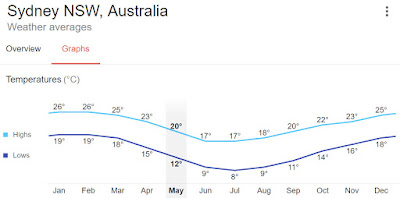## May 17, 2019

### Spline Interpolation of Monthly Data

Posted by Jason Lillywhite

Some time-varying data we use for our models is in the form of averages for time periods that are longer than the simulation time step. This can present some challenges if your model is sensitive to large jumps in the data as the simulation walks through time. For example, monthly average values in a daily simulation model will output values that are constant for all the days of each month and jump in between them. If you feel like this is not sufficient for your modeling needs, you can use linear or spline interpolation to transition smoothly between months. In this blog post, I walk through some examples that use spline interpolation to help you see how it might be of use to you and your applications.
The first example I will demonstrate is a simple model that has monthly average temperature values measured in Sydney Australia as input. I obtained the data from a quick google search:Source: NOAA
I entered these values into a GoldSim Data element with a vector [Months] data type.

The simulation runs on a daily time step. Below is a plot showing the output of these monthly values. As you can see, the temperature jumps at the change of each month because the values are held constant for each month. These kinds of jumps can easily cause headaches for models with sensitivity to change of this inputs.

A simple solution to this problem is to linearly interpolate the values using a lookup table. If I copy these values to a lookup table with independent values as the months 1-12, then I can linearly interpolate. One trick is to create a new global model property called "Decimal_Month", which is a fractional month that can be updated each day of the simulation. The equation is.
Decimal_Month = Month + (DayOfMonth - 1) / DaysInMonth
Where "Month" is the built-in run property that signifies the month with an integer between 1 and 12. "DayOfMonth" is the current day of the month in the simulation. For example, the DayOfMonth when the simulation is on Jan 15, the output will be 15. The "DaysInMonth" output is the number of calendar days in each month of the simulation. This equation will result in a fractional month count where Jan 1 starts at 1.0 and the last day in the year (Dec 31) is 12.97. This allows us to linearly interpolate on the lookup table values linearly:
Because the values are interpolated from the time of the change, which occurs at the beginning of each month, we should shift the interpolated values over by 1/2 of the month. You can do this easily using an information delay.
The result could very well be sufficient for your modeling needs. In the case shown above, the error introduced by the linear interpolation is relatively small. However, other data series might cause significant error so caution should be used when doing this type of interpolation.

For example, in a model that simulates population growth and water demand, we have 12 seasonal demand factors that are applied to the annual total water use amount in order to estimate the monthly demands. In this case, we are looking at data obtained from a site in North America. Below is a plot showing the comparison of these values and their linear interpolation.
The problem lies in areas where there is a sharp change in direction like this plot shows in July 2019. At this point, you could underestimate the value around this change. This is where a spine interpolation can help. While still an imperfect interpolation, it will not underestimate the monthly amounts as dramatically due to the curvature of the line as it is interpolated around the sharp changes. Below is a graph showing the comparison of monthly averages and the values with the spline interpolation for a table of values with sharper differences from month to month.

The next plot shows the difference between the cumulative monthly average amounts vs. cumulative amounts from the interpolated output. As shown below, the differences are insignificant.

Interpolation of monthly values is also helpful for models that apply uncertainty. Take, for example, the above input data when applying a stochastic multiplier used to represent uncertainty. I created a stochastic element that samples a random number from a normal distribution with a mean of 1.0 and a standard deviation of 0.1 and auto-correlation of 0.9 then multiply that random number by the average flow rate and it produces the following result.

As you can see, the effect of the jumps between months is unnatural and unrealistic. Some of the transitions between months is even greater than before. The interpolated model will remedy this.

The spline interpolation can be incorporated into your model by copying the model from our Model Library. It is a method first implemented in GoldSim by Camilo Gatica of Golder Associates. This method was subsequently borrowed to build the models described above for use in daily time step models that run for a longer simulation duration.

If you are interested in learning more about the spline interpolation used in GoldSim, please have a look at both of these models:

Let us know if you have any questions about how the interpolation is done or if you have problems incorporating it into your model.